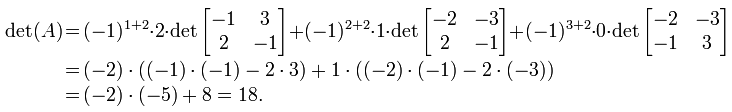## Tuesday, October 18, 2011

### The Determinant of a Matrix in NMat

The determinant will be calculated using Laplace's formula:Laplace's formula for the determinant
A - the square matrix whose determinant will be calculated
n - the size (order) of A
a - A's element at the position (i,j)
M - A's minor determined by i and j.

Example:
Let us assume that we have the following matrix:A sample 3x3 matrix
The determinant would be calculated like:The calculation of A's determinant

Our function will receive as parameters a matrix and the order of the determinant. The function shall return a number who is the matrix's determinant according to the specified order. The order should represent in most cases the size of the matrix. You can also specify a smaller than size order to compute the determinant of a minor of the matrix.

The function's definition is:
```/*
* Description:
*  Computes the determinant of a given matrix
* Parameters:
*  mat   - a pointer to the matrix
*  order - usually the size of square matrix, but it can be used
*       if you want to compute a determinant of a minor
* Returns:
*  The value of the determinant if the input is correct.
* Otherwise returns NaN.
* Preconditions:
*  @mat must not be NULL
*  @mat must be a square matrix
*  @column and @row must be smaller than @order
*  @order must be greater than 1 but smaller or equal when
*  compared to the size of the matrix
*/
real NMatrix_Determinant(const NMatrix *mat, integer order);```
The implementation of the function is:
```real NMatrix_Determinant(const NMatrix *mat, integer order)
{
integer i = 0 ,j = 0 ,k = 0 ,l = 0;
real det = 0.0;
NMatrix *minor = NULL;
if((mat->rows == mat->columns) && (order>=1) && (order<=mat->rows))
{
if(order==1)
{
det = mat->data;
}
else if(order==2)
{
det = (mat->data * mat->data) -
(mat->data * mat->data);
}
else
{
for (k=0 ; k<order ; k++)
{
minor = NMatrix_Create(order,order);
for(i=1 ; i<order ; i++)
{
l = 0;
for (j=0;j<order;j++)
{
if (j != k)
{
minor->data[i-1][l] = mat->data[i][j];
l++;
}
}
}
det += pow(-1.0,k) * mat->data[k]
* NMatrix_Determinant(minor,order-1);
minor = NMatrix_Destroy(minor);
}
}
}
else
{
det = NAN;
}
return det;
}```
Example:
```#include<stdio.h>
#include"NMatrix.h"

void PrintMatrix(NMatrix *mat)
{
integer i = 0, j = 0;
for (i = 0; i < mat->rows; i++)
{
for (j = 0; j < mat->columns; j++)
{
printf("%f ", mat->data[i][j]);
}
putchar('\n');
}
putchar('\n');
}

int main(int argc, char *argv[])
{
real det = 0;
NMatrix *mat = NULL;
/*Creates and initializes the matrix*/
mat = NMatrix_Create(3, 3);
mat->data=-2;
mat->data=2;
mat->data=-3;
mat->data=-1;
mat->data=1;
mat->data=3;
mat->data=2;
mat->data=0;
mat->data=-1;
/*Prints the matrix*/
puts("Matrix: ");
PrintMatrix(mat);
/*Computes the determinant of the matrix*/
puts("Determinant of the matrix: ");
det = NMatrix_Determinant(mat, 3);
printf("%2.1f\n", det);
/*Computes the determinant of a minor*/
puts("Determinant of a minor (M33): ");
det = NMatrix_Determinant(mat, 2);
printf("%2.1f", det);
return 0;
}
/*:
Matrix:
-2.000000 2.000000 -3.000000
-1.000000 1.000000 3.000000
2.000000 0.000000 -1.000000

Determinant of the matrix:
18.0
Determinant of a minor (M33):
0.0
*/```

1.What is this order value you mention, where does it come from, what does it mean, and how do you know it's value to input it?

1.Here's a link that explains the mathematical notions behind the order of a matrix:
http://www.mathebook.net/dict/odict/omatrix.htm

To compute the determinant of a matrix, the matrix needs to be square, so the order can be specified by using only one parameter.

Also,the order needs to specified as a parameter because the function is recursive and it calculates the matrix's determinant by decomposing the given matrix into minors and calculating their determinants.

For example, if you have a 3x3 matrix and you want to calculate its determinant you will need to compute the determinants of the 2x2 minors of the given matrix.

In the future, I will probably improve the article and provide better mathematical insight, but in the meantime you can check up Wikipedia's entry on determinants and how a determinant can be calculated using decomposition:
http://en.wikipedia.org/wiki/Determinant

So, the main idea is if you have 3x3 matrix and want its determinant calculated you will call the function as:
myDet = NMatrix_Determinant(myMatrix,3)

Got a question regarding something in the article? Leave me a comment and I will get back at you as soon as I can!